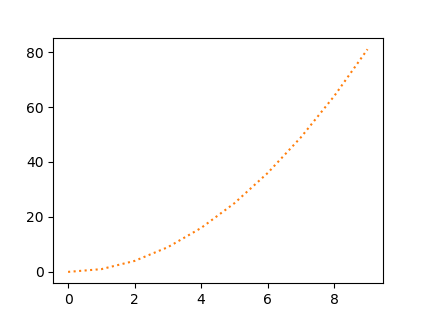#### matplotlib.pyplot做折线图的时候，显示为虚线，或者点划线？

统计/机器学习 数据可视化 Python    浏览次数：11226        分享

0luzehao   2018-08-03 09:41

##### 2个回答
4

import matplotlib.pyplot as plt
x = list(range(10))
y = [i*i for i in x]
plt.plot(x, y, linestyle=':')
plt.show()SofaSofa数据科学社区DS面试题库 DS面经nlceyes   2018-08-03 15:56

1

虚线
plt.plot(x, y, ls=':')

plt.plot(x, y, ls='--')

plt.plot(x, y, ls='-.')

SofaSofa数据科学社区DS面试题库 DS面经kym1990   2020-05-27 00:05相关主题